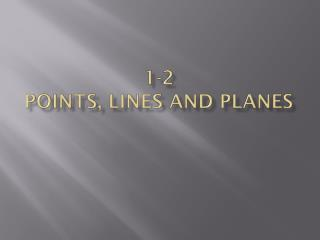DownloadDownload Presentation1-2 Points, Lines and Planes

# 1-2 Points, Lines and Planes

Download Presentation## 1-2 Points, Lines and Planes

- - - - - - - - - - - - - - - - - - - - - - - - - - - E N D - - - - - - - - - - - - - - - - - - - - - - - - - - -
##### Presentation Transcript

1. 1-2Points, Lines and Planes

2. What is geometry? What did the acorn say after it grew up? Gee, I’m a tree Geo:metry: Definition: A statement that explains the meaning of a word or phrase Format for notes: (Optional method) Earth Measure

3. Building BlocksUndefined terms • Point • Most basic building block of geometry • It has no size, only location • Notation: Capital Letter • Line • A straight, continuous arrangement of infinitely many points • Infinite length but no thickness • Extends forever in two directions • Notation: Naming any two points on line with line symbol above • Plane • Length and width but no thickness. • Like a flat surface that extends infinitely along its length and width. • Notation: Script Capital Letter P B A P

4. More Terms F E P R • Collinear • On the same line • Coplanar • On the same plane • Line Segment (segment) • Two points called endpoints of the segment and all the points between them that are collinear with the two points. • Notation: • Length: D Q B 5 cm A Endpoints

5. B More Terms A D • Congruent Segments • Two segments with the same lengths • Symbol: • Notation: • Marking figures • Midpoint • A point that divides the segment into two congruent segments C Midpoint O N M

6. …aaaaand more… • Ray • A part of a line that begins at a point and extends infinitely in one direction. • Notation: A D • Opposite Rays • Two rays that share the same endpoint and form a line. • Notation: A C and B

7. Examples 5. Name congruent segments 1. Name a line 3. Name a ray 2. Name a line segment 4. Name a midpoint K A D L B F M C N

8. A postulate or axiom is an accepted statement of fact. Postulate 1-1 Through any two points there is exactly one line. Line t passes through points A and B. Line t is the only line that passes through both points. B p. 16: 9-49 odd, 53-59 A Postulate 1-2 If two distinct lines intersect, then they intersect in exactly one point. t A B C and intersect at point C. E D